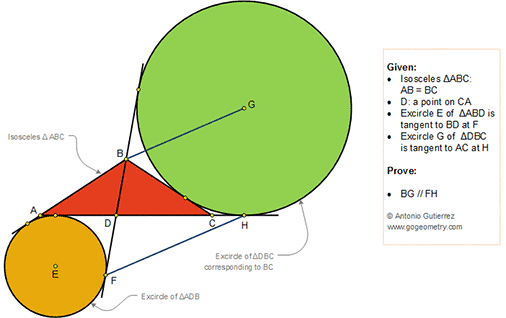## Thursday, August 9, 2018

### Geometry Problem 1376: Isosceles Triangle, Interior Cevian, Excircles, Tangency Points, Parallel Lines

Geometry Problem. Post your solution in the comment box below.
Level: Mathematics Education, High School, Honors Geometry, College.

Details: Click on the figure below.#### 1 comment:

1.https://photos.app.goo.gl/51ioXWMypPWvkQf49

Extend HG and FDB, intersect at I;

Draw BU, GT as in the photo.

Line BAP = BDF;
So BP = BD+DF =BD+DX; (1)
Line DBT=DCH
DB+BT=DC+CH ;
So DB+BY=DC+CH ;
So DB= DC+CH-BY (2)
From (1), (2);
BP = BF= DX + DC+CH-BY =DX+DC-(BC-YC)+CH=XC-BC + 2CH= AX –AX+XC-BC+2CH
=AC-AX- BC+2CH= AC-AX- BA+2CH =AC+2CH-BP
So BP=1/2 AC+CH= BU
BP=BF=BU;
Because TGxBI = BUxGI;
So GHXBI =BFxGI;
So BI/BF = GI/GH
So BG//FH